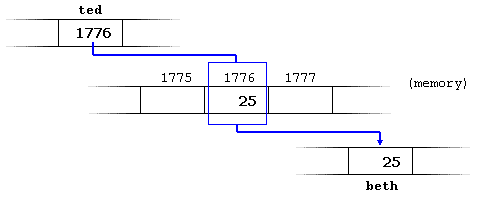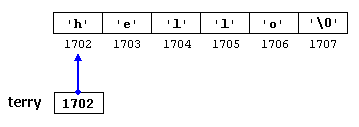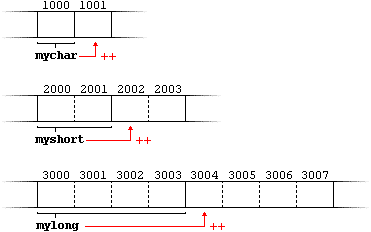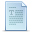# Pointers

We have already seen how variables are seen as memory cells that can be accessed using their identifiers. This way we did not have to care about the physical location of our data within memory, we simply used its identifier whenever we wanted to refer to our variable.

The memory of your computer can be imagined as a succession of memory cells, each one of the minimal size that computers manage (one byte). These single-byte memory cells are numbered in a consecutive way, so as, within any block of memory, every cell has the same number as the previous one plus one.

This way, each cell can be easily located in the memory because it has a unique address and all the memory cells follow a successive pattern. For example, if we are looking for cell 1776 we know that it is going to be right between cells 1775 and 1777, exactly one thousand cells after 776 and exactly one thousand cells before cell 2776.

### Reference operator (&)

As soon as we declare a variable, the amount of memory needed is assigned for it at a specific location in memory (its memory address). We generally do not actively decide the exact location of the variable within the panel of cells that we have imagined the memory to be - Fortunately, that is a task automatically performed by the operating system during runtime. However, in some cases we may be interested in knowing the address where our variable is being stored during runtime in order to operate with relative positions to it.

The address that locates a variable within memory is what we call a reference to that variable. This reference to a variable can be obtained by preceding the identifier of a variable with an ampersand sign (&), known as reference operator, and which can be literally translated as "address of". For example:

 `` `` ``ted = &andy;``

This would assign to ted the address of variable andy, since when preceding the name of the variable andy with the reference operator (&) we are no longer talking about the content of the variable itself, but about its reference (i.e., its address in memory).

From now on we are going to assume that andy is placed during runtime in the memory address 1776. This number (1776) is just an arbitrary assumption we are inventing right now in order to help clarify some concepts in this tutorial, but in reality, we cannot know before runtime the real value the address of a variable will have in memory.

Consider the following code fragment:

 ``123`` ``````andy = 25; fred = andy; ted = &andy; ``````

The values contained in each variable after the execution of this, are shown in the following diagram:First, we have assigned the value 25 to andy (a variable whose address in memory we have assumed to be 1776).

The second statement copied to fred the content of variable andy (which is 25). This is a standard assignment operation, as we have done so many times before.

Finally, the third statement copies to ted not the value contained in andy but a reference to it (i.e., its address, which we have assumed to be 1776). The reason is that in this third assignment operation we have preceded the identifier andy with the reference operator (&), so we were no longer referring to the value of andy but to its reference (its address in memory).

The variable that stores the reference to another variable (like ted in the previous example) is what we call a pointer. Pointers are a very powerful feature of the C++ language that has many uses in advanced programming. Farther ahead, we will see how this type of variable is used and declared.

### Dereference operator (*)

We have just seen that a variable which stores a reference to another variable is called a pointer. Pointers are said to "point to" the variable whose reference they store.

Using a pointer we can directly access the value stored in the variable which it points to. To do this, we simply have to precede the pointer's identifier with an asterisk (*), which acts as dereference operator and that can be literally translated to "value pointed by".

Therefore, following with the values of the previous example, if we write:

 `` `` ``beth = *ted;``

(that we could read as: "beth equal to value pointed by ted") beth would take the value 25, since ted is 1776, and the value pointed by 1776 is 25.You must clearly differentiate that the expression ted refers to the value 1776, while *ted (with an asterisk * preceding the identifier) refers to the value stored at address 1776, which in this case is 25. Notice the difference of including or not including the dereference operator (I have included an explanatory commentary of how each of these two expressions could be read):

 ``12`` ``````beth = ted; // beth equal to ted ( 1776 ) beth = *ted; // beth equal to value pointed by ted ( 25 ) ``````

Notice the difference between the reference and dereference operators:
• & is the reference operator and can be read as "address of"
• * is the dereference operator and can be read as "value pointed by"
Thus, they have complementary (or opposite) meanings. A variable referenced with & can be dereferenced with *.

Earlier we performed the following two assignment operations:

 ``12`` ``````andy = 25; ted = &andy;``````

Right after these two statements, all of the following expressions would give true as result:

 ``1234`` ``````andy == 25 &andy == 1776 ted == 1776 *ted == 25``````

The first expression is quite clear considering that the assignment operation performed on andy was andy=25. The second one uses the reference operator (&), which returns the address of variable andy, which we assumed it to have a value of 1776. The third one is somewhat obvious since the second expression was true and the assignment operation performed on ted was ted=&andy. The fourth expression uses the dereference operator (*) that, as we have just seen, can be read as "value pointed by", and the value pointed by ted is indeed 25.

So, after all that, you may also infer that for as long as the address pointed by ted remains unchanged the following expression will also be true:

 `` `` ``*ted == andy``

### Declaring variables of pointer types

Due to the ability of a pointer to directly refer to the value that it points to, it becomes necessary to specify in its declaration which data type a pointer is going to point to. It is not the same thing to point to a char as to point to an int or a float.

The declaration of pointers follows this format:

type * name;

where type is the data type of the value that the pointer is intended to point to. This type is not the type of the pointer itself! but the type of the data the pointer points to. For example:

 ``123`` ``````int * number; char * character; float * greatnumber;``````

These are three declarations of pointers. Each one is intended to point to a different data type, but in fact all of them are pointers and all of them will occupy the same amount of space in memory (the size in memory of a pointer depends on the platform where the code is going to run). Nevertheless, the data to which they point to do not occupy the same amount of space nor are of the same type: the first one points to an int, the second one to a char and the last one to a float. Therefore, although these three example variables are all of them pointers which occupy the same size in memory, they are said to have different types: int*, char* and float* respectively, depending on the type they point to.

I want to emphasize that the asterisk sign (*) that we use when declaring a pointer only means that it is a pointer (it is part of its type compound specifier), and should not be confused with the dereference operator that we have seen a bit earlier, but which is also written with an asterisk (*). They are simply two different things represented with the same sign.

Now have a look at this code:

 ``1234567891011121314151617`` ``````// my first pointer #include using namespace std; int main () { int firstvalue, secondvalue; int * mypointer; mypointer = &firstvalue; *mypointer = 10; mypointer = &secondvalue; *mypointer = 20; cout << "firstvalue is " << firstvalue << endl; cout << "secondvalue is " << secondvalue << endl; return 0; }`````` ```firstvalue is 10 secondvalue is 20```

Notice that even though we have never directly set a value to either firstvalue or secondvalue, both end up with a value set indirectly through the use of mypointer. This is the procedure:

First, we have assigned as value of mypointer a reference to firstvalue using the reference operator (&). And then we have assigned the value 10 to the memory location pointed by mypointer, that because at this moment is pointing to the memory location of firstvalue, this in fact modifies the value of firstvalue.

In order to demonstrate that a pointer may take several different values during the same program I have repeated the process with secondvalue and that same pointer, mypointer.

Here is an example a little bit more elaborated:

 ``1234567891011121314151617181920`` ``````// more pointers #include using namespace std; int main () { int firstvalue = 5, secondvalue = 15; int * p1, * p2; p1 = &firstvalue; // p1 = address of firstvalue p2 = &secondvalue; // p2 = address of secondvalue *p1 = 10; // value pointed by p1 = 10 *p2 = *p1; // value pointed by p2 = value pointed by p1 p1 = p2; // p1 = p2 (value of pointer is copied) *p1 = 20; // value pointed by p1 = 20 cout << "firstvalue is " << firstvalue << endl; cout << "secondvalue is " << secondvalue << endl; return 0; }`````` ```firstvalue is 10 secondvalue is 20```

I have included as a comment on each line how the code can be read: ampersand (&) as "address of" and asterisk (*) as "value pointed by".

Notice that there are expressions with pointers p1 and p2, both with and without dereference operator (*). The meaning of an expression using the dereference operator (*) is very different from one that does not: When this operator precedes the pointer name, the expression refers to the value being pointed, while when a pointer name appears without this operator, it refers to the value of the pointer itself (i.e. the address of what the pointer is pointing to).

Another thing that may call your attention is the line:

 `` `` ``int * p1, * p2;``

This declares the two pointers used in the previous example. But notice that there is an asterisk (*) for each pointer, in order for both to have type int* (pointer to int).

Otherwise, the type for the second variable declared in that line would have been int (and not int*) because of precedence relationships. If we had written:

 `` `` ``int * p1, p2;``

p1 would indeed have int* type, but p2 would have type int (spaces do not matter at all for this purpose). This is due to operator precedence rules. But anyway, simply remembering that you have to put one asterisk per pointer is enough for most pointer users.

### Pointers and arrays

The concept of array is very much bound to the one of pointer. In fact, the identifier of an array is equivalent to the address of its first element, as a pointer is equivalent to the address of the first element that it points to, so in fact they are the same concept. For example, supposing these two declarations:

 ``12`` ``````int numbers ; int * p;``````

The following assignment operation would be valid:

 `` `` ``p = numbers; ``

After that, p and numbers would be equivalent and would have the same properties. The only difference is that we could change the value of pointer p by another one, whereas numbers will always point to the first of the 20 elements of type int with which it was defined. Therefore, unlike p, which is an ordinary pointer, numbers is an array, and an array can be considered a constant pointer. Therefore, the following allocation would not be valid:

 `` `` ``numbers = p;``

Because numbers is an array, so it operates as a constant pointer, and we cannot assign values to constants.

Due to the characteristics of variables, all expressions that include pointers in the following example are perfectly valid:

 ``1234567891011121314151617`` ``````// more pointers #include using namespace std; int main () { int numbers; int * p; p = numbers; *p = 10; p++; *p = 20; p = &numbers; *p = 30; p = numbers + 3; *p = 40; p = numbers; *(p+4) = 50; for (int n=0; n<5; n++) cout << numbers[n] << ", "; return 0; }`````` `10, 20, 30, 40, 50, `

In the chapter about arrays we used brackets ([]) several times in order to specify the index of an element of the array to which we wanted to refer. Well, these bracket sign operators [] are also a dereference operator known as offset operator. They dereference the variable they follow just as * does, but they also add the number between brackets to the address being dereferenced. For example:

 ``12`` ``````a = 0; // a [offset of 5] = 0 *(a+5) = 0; // pointed by (a+5) = 0 ``````

These two expressions are equivalent and valid both if a is a pointer or if a is an array.

### Pointer initialization

When declaring pointers we may want to explicitly specify which variable we want them to point to:

 ``12`` ``````int number; int *tommy = &number;``````

The behavior of this code is equivalent to:

 ``123`` ``````int number; int *tommy; tommy = &number;``````

When a pointer initialization takes place we are always assigning the reference value to where the pointer points (tommy), never the value being pointed (*tommy). You must consider that at the moment of declaring a pointer, the asterisk (*) indicates only that it is a pointer, it is not the dereference operator (although both use the same sign: *). Remember, they are two different functions of one sign. Thus, we must take care not to confuse the previous code with:

 ``123`` ``````int number; int *tommy; *tommy = &number;``````

that is incorrect, and anyway would not have much sense in this case if you think about it.

As in the case of arrays, the compiler allows the special case that we want to initialize the content at which the pointer points with constants at the same moment the pointer is declared:

 `` `` ``const char * terry = "hello"; ``

In this case, memory space is reserved to contain "hello" and then a pointer to the first character of this memory block is assigned to terry. If we imagine that "hello" is stored at the memory locations that start at addresses 1702, we can represent the previous declaration as:It is important to indicate that terry contains the value 1702, and not 'h' nor "hello", although 1702 indeed is the address of both of these.

The pointer terry points to a sequence of characters and can be read as if it was an array (remember that an array is just like a constant pointer). For example, we can access the fifth element of the array with any of these two expression:

 ``12`` ``````*(terry+4) terry``````

Both expressions have a value of 'o' (the fifth element of the array).

### Pointer arithmetics

To conduct arithmetical operations on pointers is a little different than to conduct them on regular integer data types. To begin with, only addition and subtraction operations are allowed to be conducted with them, the others make no sense in the world of pointers. But both addition and subtraction have a different behavior with pointers according to the size of the data type to which they point.

When we saw the different fundamental data types, we saw that some occupy more or less space than others in the memory. For example, let's assume that in a given compiler for a specific machine, char takes 1 byte, short takes 2 bytes and long takes 4.

Suppose that we define three pointers in this compiler:

 ``123`` ``````char *mychar; short *myshort; long *mylong;``````

and that we know that they point to memory locations 1000, 2000 and 3000 respectively.

So if we write:

 ``123`` ``````mychar++; myshort++; mylong++;``````

mychar, as you may expect, would contain the value 1001. But not so obviously, myshort would contain the value 2002, and mylong would contain 3004, even though they have each been increased only once. The reason is that when adding one to a pointer we are making it to point to the following element of the same type with which it has been defined, and therefore the size in bytes of the type pointed is added to the pointer.This is applicable both when adding and subtracting any number to a pointer. It would happen exactly the same if we write:

 ``123`` ``````mychar = mychar + 1; myshort = myshort + 1; mylong = mylong + 1;``````

Both the increase (++) and decrease (--) operators have greater operator precedence than the dereference operator (*), but both have a special behavior when used as suffix (the expression is evaluated with the value it had before being increased). Therefore, the following expression may lead to confusion:

 `` `` ``*p++``

Because ++ has greater precedence than *, this expression is equivalent to *(p++). Therefore, what it does is to increase the value of p (so it now points to the next element), but because ++ is used as postfix the whole expression is evaluated as the value pointed by the original reference (the address the pointer pointed to before being increased).

Notice the difference with:

(*p)++
Here, the expression would have been evaluated as the value pointed by p increased by one. The value of p (the pointer itself) would not be modified (what is being modified is what it is being pointed to by this pointer).

If we write:

 `` `` ``*p++ = *q++; ``

Because ++ has a higher precedence than *, both p and q are increased, but because both increase operators (++) are used as postfix and not prefix, the value assigned to *p is *q before both p and q are increased. And then both are increased. It would be roughly equivalent to:

 ``123`` ``````*p = *q; ++p; ++q;``````

Like always, I recommend you to use parentheses () in order to avoid unexpected results and to give more legibility to the code.

### Pointers to pointers

C++ allows the use of pointers that point to pointers, that these, in its turn, point to data (or even to other pointers). In order to do that, we only need to add an asterisk (*) for each level of reference in their declarations:

 ``123456`` ``````char a; char * b; char ** c; a = 'z'; b = &a; c = &b;``````

This, supposing the randomly chosen memory locations for each variable of 7230, 8092 and 10502, could be represented as:The value of each variable is written inside each cell; under the cells are their respective addresses in memory.

The new thing in this example is variable c, which can be used in three different levels of indirection, each one of them would correspond to a different value:

• c has type char** and a value of 8092
• *c has type char* and a value of 7230
• **c has type char and a value of 'z'

### void pointers

The void type of pointer is a special type of pointer. In C++, void represents the absence of type, so void pointers are pointers that point to a value that has no type (and thus also an undetermined length and undetermined dereference properties).

This allows void pointers to point to any data type, from an integer value or a float to a string of characters. But in exchange they have a great limitation: the data pointed by them cannot be directly dereferenced (which is logical, since we have no type to dereference to), and for that reason we will always have to cast the address in the void pointer to some other pointer type that points to a concrete data type before dereferencing it.

One of its uses may be to pass generic parameters to a function:

 ``123456789101112131415161718192021`` ``````// increaser #include using namespace std; void increase (void* data, int psize) { if ( psize == sizeof(char) ) { char* pchar; pchar=(char*)data; ++(*pchar); } else if (psize == sizeof(int) ) { int* pint; pint=(int*)data; ++(*pint); } } int main () { char a = 'x'; int b = 1602; increase (&a,sizeof(a)); increase (&b,sizeof(b)); cout << a << ", " << b << endl; return 0; }`````` `y, 1603`

sizeof is an operator integrated in the C++ language that returns the size in bytes of its parameter. For non-dynamic data types this value is a constant. Therefore, for example, sizeof(char) is 1, because char type is one byte long.

### Null pointer

A null pointer is a regular pointer of any pointer type which has a special value that indicates that it is not pointing to any valid reference or memory address. This value is the result of type-casting the integer value zero to any pointer type.

 ``12`` ``````int * p; p = 0; // p has a null pointer value ``````

Do not confuse null pointers with void pointers. A null pointer is a value that any pointer may take to represent that it is pointing to "nowhere", while a void pointer is a special type of pointer that can point to somewhere without a specific type. One refers to the value stored in the pointer itself and the other to the type of data it points to.

### Pointers to functions

C++ allows operations with pointers to functions. The typical use of this is for passing a function as an argument to another function, since these cannot be passed dereferenced. In order to declare a pointer to a function we have to declare it like the prototype of the function except that the name of the function is enclosed between parentheses () and an asterisk (*) is inserted before the name:

 ``123456789101112131415161718192021222324252627`` ``````// pointer to functions #include using namespace std; int addition (int a, int b) { return (a+b); } int subtraction (int a, int b) { return (a-b); } int operation (int x, int y, int (*functocall)(int,int)) { int g; g = (*functocall)(x,y); return (g); } int main () { int m,n; int (*minus)(int,int) = subtraction; m = operation (7, 5, addition); n = operation (20, m, minus); cout <

In the example, minus is a pointer to a function that has two parameters of type int. It is immediately assigned to point to the function subtraction, all in a single line:

 `` `` ``int (* minus)(int,int) = subtraction;``Previous:Character SequencesIndexNext:Dynamic Memory